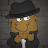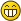Password Decoration RuleeljolotMemberPosts: 88 Threads: 16 Joined: Nov 2012 01-13-2013, 06:31 PM yeah I saw, I already commented that thread, that > 15 char only in hashcatcli is posible. and doing -a 1 with him I founded some hashed hashes with some appended words like "testing" + hash. but is very time consuming.KuciMemberPosts: 179 Threads: 13 Joined: Dec 2012 01-13-2013, 09:53 PM Very nice work, Hash-ITtheberghJunior MemberPosts: 6 Threads: 1 Joined: Jan 2013 01-14-2013, 05:48 PM Thanks man! A few hashes I have been working on for a long time got cracked thanks to your rules.Hash-ITModeratorPosts: 723 Threads: 85 Joined: Apr 2011 01-14-2013, 06:25 PM (01-13-2013, 09:53 PM)Kuci Wrote: Very nice work, Hash-ITYou're welcomethebergh Wrote:Thanks man! A few hashes I have been working on for a long time got cracked thanks to your rules. That's great !RolfТоварищ РолфPosts: 601 Threads: 18 Joined: Apr 2010 01-19-2013, 03:17 PM Rolf approves! Good work and thanks for making this public.Hash-ITModeratorPosts: 723 Threads: 85 Joined: Apr 2011 01-19-2013, 03:38 PM (01-19-2013, 03:17 PM)Rolf Wrote: Rolf approves! Good work and thanks for making this public.Thank you ! I hope you find them useful.WaffleJunior MemberPosts: 30 Threads: 7 Joined: Dec 2012 01-19-2013, 05:21 PM Nice job! I was surprised that they ran quite fast, as well! Well done.Hash-ITModeratorPosts: 723 Threads: 85 Joined: Apr 2011 01-19-2013, 06:47 PM (01-19-2013, 05:21 PM)Waffle Wrote: Nice job! I was surprised that they ran quite fast, as well! Well done. Thank youThis set of rules should be better when / if oclhashcat-plus supports longer passwords.forumheroSenior MemberPosts: 313 Threads: 44 Joined: Aug 2011 01-25-2013, 07:40 AM hmm for some reason it doesn't work for me Code:```Skipping invalid or unsupported rule in file ../rules/hashit.rule in line 1: ^O^.^o^Â°^Â¨\$O\$.\$o\$Â°\$Â¨ Skipping invalid or unsupported rule in file ../rules/hashit.rule in line 2: ^Â±^â€¡^Â±^Ã·^Â·\$Â±\$â€¡\$Â±\$Ã·\$. Skipping invalid or unsupported rule in file ../rules/hashit.rule in line 4: ^+^Â«^?^Â»^+\$+\$Â»\$?\$Â«\$+ Skipping invalid or unsupported rule in file ../rules/hashit.rule in line 5: ^O^.^o^Â°^â€¢\$O\$.\$o\$Â°\$â€¢ Skipping invalid or unsupported rule in file ../rules/hashit.rule in line 6: ^Â¨^'^Â°^?^O\$Â¨\$'\$Â°\$?\$O Skipping invalid or unsupported rule in file ../rules/hashit.rule in line 7: ^Â¨^â€˜^Â°^Âº^O\$Â¨\$â€˜\$Â°\$Âº\$O Skipping invalid or unsupported rule in file ../rules/hashit.rule in line 8: ^Â·^[^Â¦^!^Â·\$Â·\$]\$Â¦\$!\$Â· Skipping invalid or unsupported rule in file ../rules/hashit.rule in line 9: ^[^Ã·^â€¢^â€¢^(^â€”\$]\$Ã·\$â€¢\$â€¢\$â€” Skipping invalid or unsupported rule in file ../rules/hashit.rule in line 10: ^.^â€¢^`^Â¯^Â´^â€¢\$.\$â€¢\$Â´\$Â¯\$`\$â€¢ Skipping invalid or unsupported rule in file ../rules/hashit.rule in line 11: ^[^Ã·^Â·^â€¢^(^â€”\$]\$Ã·\$Â·\$â€¢\$)\$â€” Skipping invalid or unsupported rule in file ../rules/hashit.rule in line 12: ^_^Â¯^_^Â¯^_^Â¯\$_\$Â¯\$_\$Â¯\$_\$Â¯ Skipping invalid or unsupported rule in file ../rules/hashit.rule in line 13: ^Â°^l^|^|^l^Â°\$Â°\$l\$|\$|\$l\$Â° Skipping invalid or unsupported rule in file ../rules/hashit.rule in line 14: ^Â»^â€¢^`^Â¯^Â´^â€¢\$Â«\$â€¢\$Â´\$Â¯\$`\$â€¢ Skipping invalid or unsupported rule in file ../rules/hashit.rule in line 15: ^â€¢^â€¢^`^Â¯^Â°^â€¢\$â€¢\$â€¢\$Â´\$Â¯\$Â°\$â€¢ Skipping invalid or unsupported rule in file ../rules/hashit.rule in line 16: ^)^_^.^Â·^`^Â¯^(\$(\$Â¯\$`\$Â·\$.\$_\$) Skipping invalid or unsupported rule in file ../rules/hashit.rule in line 17: ^)^_^.^â€¢^`^Â¯^(\$(\$Â¯\$`\$â€¢\$.\$_\$) Skipping invalid or unsupported rule in file ../rules/hashit.rule in line 18: ^+^*^Â¨^^^Â¨^*^+\$+\$*\$Â¨\$^\$Â¨\$*\$+ Skipping invalid or unsupported rule in file ../rules/hashit.rule in line 20: ^Â·^Ã¯^Â¡^Ã·^Â¡^Ã¯^Â·\$Â·\$Ã¯\$Â¡\$Ã·\$Â¡\$Ã¯\$Â· Skipping invalid or unsupported rule in file ../rules/hashit.rule in line 21: ^*^Â¤^[^`^(^Ã·^Â¤^â€”\$*\$Â¤\$]\$Â´\$)\$Ã·\$Â¤\$â€” Skipping invalid or unsupported rule in file ../rules/hashit.rule in line 22: ^>^-^Â·^`^Â¯^Â´^Â·^.\$<\$-\$Â·\$Â´\$Â¯\$`\$Â·\$. Cannot convert rule for use on GPU in file ../rules/hashit.rule in line 23: ^[^^^-^-^ ^-^ ^-\$]\$^\$-\$-\$ \$-\$ \$- Skipping invalid or unsupported rule in file ../rules/hashit.rule in line 24: ^]^^^-^-^Â¤^-^ ^-\$[\$^\$-\$-\$Â¤\$-\$ \$- Skipping invalid or unsupported rule in file ../rules/hashit.rule in line 25: ^]^Â©^[^]^Â§^Â®^~^Â©\$[\$Â©\$]\$[\$Â§\$Â®\$~\$Â© Skipping invalid or unsupported rule in file ../rules/hashit.rule in line 26: ^Â¤^[^Ã—^`^(^Â¤^Â·^Â·\$Â¤\$]\$Ã—\$Â´\$)\$Â¤\$Â·\$Â· Skipping invalid or unsupported rule in file ../rules/hashit.rule in line 27: ^Â¤^[^Ã—^`^(^Â¤^â€¢^â€¢\$Â¤\$]\$Ã—\$Â´\$)\$Â¤\$â€¢\$â€¢ Skipping invalid or unsupported rule in file ../rules/hashit.rule in line 28: ^Â¦^Â¦^Â¦^Â¦^Â¦^Â¦^Â¦^Â¦\$Â¦\$Â¦\$Â¦\$Â¦\$Â¦\$Â¦\$Â¦\$Â¦ Skipping invalid or unsupported rule in file ../rules/hashit.rule in line 29: ^â€¢^-^(^â€¢^â€“^â€“^â€“^â€“\$â€¢\$-\$)\$â€¢\$â€“\$â€“\$â€“\$â€“ Skipping invalid or unsupported rule in file ../rules/hashit.rule in line 30: ^â€¢^.^_^.^â€¢^Â´^Â¯^(\$â€¢\$.\$_\$.\$â€¢\$Â´\$Â¯\$) Cannot convert rule for use on GPU in file ../rules/hashit.rule in line 31: ^[^^^-^-^ ^-^ ^-\$]\$ \$^\$-\$-\$ \$-\$ \$- Skipping invalid or unsupported rule in file ../rules/hashit.rule in line 32: ^[^Â·^`^(^)^Â´^.^Â¸\$]\$Â·\$Â´\$)\$(\$`\$ \$.\$Â¸ Cannot convert rule for use on GPU in file ../rules/hashit.rule in line 33: ^_^+^(^<^.^.^|^.^.\$_\$+\$>\$.\$.\$|\$.\$. Skipping invalid or unsupported rule in file ../rules/hashit.rule in line 34: ^)^Â¯^Â´^Â·^Â¸^â€¢^`^Â¯^(\$(\$Â¯\$`\$Â·\$Â¸\$â€¢\$Â´\$Â¯\$) Skipping invalid or unsupported rule in file ../rules/hashit.rule in line 35: ^-^Â·^=^Â«^â€¡^Â»^=^Â·^-\$-\$Â·\$=\$Â»\$â€¡\$Â«\$=\$Â·\$- Skipping invalid or unsupported rule in file ../rules/hashit.rule in line 36: ^.^o^0^Ã—^X^Ã—^0^o^.\$.\$o\$0\$Ã—\$X\$Ã—\$0\$o\$. Skipping invalid or unsupported rule in file ../rules/hashit.rule in line 37: ^[^Â·^.^_^.^Â·^`^Â¯^(\$]\$Â·\$.\$_\$.\$Â·\$Â´\$Â¯\$) Skipping invalid or unsupported rule in file ../rules/hashit.rule in line 38: ^[^â€¢^.^_^.^â€¢^`^Â¯^(\$]\$â€¢\$.\$_\$.\$â€¢\$Â´\$Â¯\$) Skipping invalid or unsupported rule in file ../rules/hashit.rule in line 39: ^]^Â§^[^]^Â®^~^Â©^~^Â®\$[\$Â§\$]\$[\$Â®\$~\$Â©\$~\$Â® Skipping invalid or unsupported rule in file ../rules/hashit.rule in line 40: ^Â»^â€¢^Â´^Âº^Â´^â€¢^â€¢^]^â€¢\$Â«\$â€¢\$Â´\$Âº\$Â´\$â€¢\$â€¢\$[\$â€¢ Skipping invalid or unsupported rule in file ../rules/hashit.rule in line 41: ^Â»^â€¢^`^Â¯^Â´^â€¢^.^â€¢^Ã·^Ã—\$Â«\$â€¢\$Â´\$Â¯\$`\$â€¢\$.\$â€¢\$Ã·\$Ã— Skipping invalid or unsupported rule in file ../rules/hashit.rule in line 42: ^Ã—^?^Â°^"^`^?^"^Â°^?^Ã—\$Ã—\$?\$Â°\$"\$?\$`\$"\$Â°\$?\$Ã— Skipping invalid or unsupported rule in file ../rules/hashit.rule in line 43: ^Ã—^Âº^Â°^â€^`^Ëœ^â€^Â°^Âº^Ã—\$Ã—\$Âº\$Â°\$â€\$`\$Ëœ\$â€\$Â°\$Âº\$Ã— Skipping invalid or unsupported rule in file ../rules/hashit.rule in line 44: ^Ã—^Âº^Â°^â€^`^Ëœ^â€^Â°^Âº^Ã—\$Ã—\$Âº\$Â°\$â€\$Ëœ\$`\$â€\$Â°\$Âº\$Ã— Skipping invalid or unsupported rule in file ../rules/hashit.rule in line 45: ^)^Ã—^`^Â¯^Â´^v^^^Â·^Â·^ ^Â·\$(\$Ã—\$Â´\$Â¯\$`\$v\$^\$Â·\$Â·\$ \$Â· Skipping invalid or unsupported rule in file ../rules/hashit.rule in line 46: ^?^Â·^.^Â·^`^Â¯^Â´^Â·^.^Â·^?\$?\$Â·\$.\$Â·\$Â´\$Â¯\$`\$Â·\$.\$Â·\$? Skipping invalid or unsupported rule in file ../rules/hashit.rule in line 47: ^Â¯^Â´^â€¢^.^,^Â¸^Â¸^,^.^â€¢^`\$Â¯\$`\$â€¢\$.\$,\$Â¸\$Â¸\$,\$.\$â€¢\$Â´ Skipping invalid or unsupported rule in file ../rules/hashit.rule in line 48: ^Â»^)^Â·^`^Â¯^Â´^Â·^.^Â·^Ã·^Ã—\$Â«\$(\$Â·\$Â´\$Â¯\$`\$Â·\$.\$Â·\$Ã·\$Ã— Skipping invalid or unsupported rule in file ../rules/hashit.rule in line 49: ^Â»^)^â€¢^`^Â¯^Â´^â€¢^.^â€¢^Ã·^Ã—\$Â«\$(\$â€¢\$Â´\$Â¯\$`\$â€¢\$.\$â€¢\$Ã·\$Ã— Skipping invalid or unsupported rule in file ../rules/hashit.rule in line 50: ^Â»^-^)^Â¯^Â´^v^`^Â¯^(^-^Â»\$Â»\$-\$(\$Â¯\$`\$v\$Â´\$Â¯\$)\$-\$Â» Skipping invalid or unsupported rule in file ../rules/hashit.rule in line 51: ^â€¢^.^_^.^Â·^Â°^Â¯^(^(^?^â€¢\$â€¢\$.\$_\$.\$Â·\$Â°\$Â¯\$)\$)\$?\$â€¢ Skipping invalid or unsupported rule in file ../rules/hashit.rule in line 52: ^â€¢^Â·^.^Â·^`^Â¯^Â´^Â·^.^Â·^â€¢\$â€¢\$Â·\$.\$Â·\$Â´\$Â¯\$`\$Â·\$.\$Â·\$â€¢ Skipping invalid or unsupported rule in file ../rules/hashit.rule in line 53: ^â€¢^â€¢^.^â€¢^`^Â¯^Â´^â€¢^.^â€¢^â€¢\$â€¢\$â€¢\$.\$â€¢\$Â´\$Â¯\$`\$â€¢\$.\$â€¢\$â€¢ Skipping invalid or unsupported rule in file ../rules/hashit.rule in line 54: ^(^)^F^(^Â°^`^Â¯^(^*^Â¤^Â°^â€¢\$)\$(\$F\$)\$Â°\$Â´\$Â¯\$)\$*\$Â¤\$Â°\$â€¢ Skipping invalid or unsupported rule in file ../rules/hashit.rule in line 55: ^Â¨^Ëœ^Ë†^â€^Â°^Â¹^~^â€¢^-^.^â€ž^Â¸\$Â¨\$Ëœ\$Ë†\$â€\$Â°\$Â¹\$~\$â€¢\$-\$.\$â€ž\$Â¸ Skipping invalid or unsupported rule in file ../rules/hashit.rule in line 56: ^Â»^-^-^-^-^-^-^â€¢^{^I^|^]\$Â«\$-\$-\$-\$-\$-\$-\$â€¢\$}\$I\$|\$[ Skipping invalid or unsupported rule in file ../rules/hashit.rule in line 57: ^]^.^~^*^Â°^o^Â°^Â°^o^Â°^Â°^o\$[\$.\$~\$*\$ \$Â°\$o\$Â°\$Â°\$o\$Â°\$Â°\$o Skipping invalid or unsupported rule in file ../rules/hashit.rule in line 58: ^Ã—^.^.^â€¢^Ã·^Â°^'^Â¨^Â´^â€¢^.^Â§\$Ã—\$,\$.\$â€¢\$Â´\$Â¨\$'\$Â°\$Ã·\$â€¢\$.\$.\$Â§ Skipping invalid or unsupported rule in file ../rules/hashit.rule in line 59: ^~^?^Â·^*^'^Â¨^Â¯^Â¨^'^*^~^?^-\$~\$?\$*\$'\$Â¨\$Â¯\$Â¨\$'\$*\$Â·\$~\$?\$- Skipping invalid or unsupported rule in file ../rules/hashit.rule in line 60: ^Â¸^,^Ã¸^Â¤^Âº^Â°^`^Â°^Âº^Â¤^Ã¸^,^Â¸\$Â¸\$,\$Ã¸\$Â¤\$Âº\$Â°\$`\$Â°\$Âº\$Â¤\$Ã¸\$,\$Â¸ Skipping invalid or unsupported rule in file ../rules/hashit.rule in line 61: ^*^`^Â¯^Â´^*^.^Â¸^Â¸^.^*^`^Â¯^Â´^*\$*\$Â´\$Â¯\$`\$*\$.\$Â¸\$Â¸\$.\$*\$Â´\$Â¯\$`\$* Skipping invalid or unsupported rule in file ../rules/hashit.rule in line 62: ^|^!^Â¤^*^'^~^`^`^~^'^*^Â¤^!^|\$|\$!\$Â¤\$*\$'\$~\$`\$`\$~\$'\$*\$Â¤\$!\$| Skipping invalid or unsupported rule in file ../rules/hashit.rule in line 63: ^*^*^O^~^Â°^'^Â¨^Â¤^Â¦^Â¤^Â¨^'^Â°^~^O\$*\$*\$O\$~\$Â°\$'\$Â¨\$Â¤\$Â¦\$Â¤\$Â¨\$'\$Â°\$~\$O Cannot convert rule for use on GPU in file ../rules/hashit.rule in line 64: ^.^_^|^.^>^)^+^_^+^(^<^.^|^_^.\$.\$_\$|\$.\$<\$(\$+\$_\$+\$)\$>\$.\$|\$_\$. Skipping invalid or unsupported rule in file ../rules/hashit.rule in line 65: ^Â°^Â¤^*^)^Â¯^Â´^?^`^Â¯^(^*^Â¤^Â°^â€¢^.\$Â°\$Â¤\$*\$(\$Â¯\$Â´\$?\$`\$Â¯\$)\$*\$Â¤\$Â°\$â€¢\$. Skipping invalid or unsupported rule in file ../rules/hashit.rule in line 66: ^?^?^Â°^`^Â°^Âº^Â¤^Ã¸^,^Â¸^Â¸^,^?^?^?\$?\$?\$ \$,\$Â¸\$Â¸\$,\$Ã¸\$Â¤\$Âº\$Â°\$`\$Â°\$?\$?\$? Skipping invalid or unsupported rule in file ../rules/hashit.rule in line 67: ^'^*^~^-^.^,^Â¸^Â¸^.^-^~^Â·^*^'^Â¨^Â¯\$Â¯\$Â¨\$'\$*\$Â·\$~\$-\$.\$Â¸\$Â¸\$,\$.\$-\$~\$*\$' Skipping invalid or unsupported rule in file ../rules/hashit.rule in line 68: ^'^*^~^-^.^,^Â¸^Â¸^.^-^~^â€¢^*^'^Â¨^Â¯\$Â¯\$Â¨\$'\$*\$â€¢\$~\$-\$.\$Â¸\$Â¸\$,\$.\$-\$~\$*\$' Skipping invalid or unsupported rule in file ../rules/hashit.rule in line 69: ^.^Â·^`^Â¯^Â´^Â·^.^<^>^)^)^)^)^Âº^<\$Â¸\$.\$Â·\$Â´\$Â¯\$`\$Â·\$.\$Â¸\$>\$<\$(\$(\$(\$(\$Âº\$> Skipping invalid or unsupported rule in file ../rules/hashit.rule in line 70: ^.^Â·^`^Â¯^Â´^Â·^.^<^>^Âº^(^(^(^(^<\$Â¸\$.\$Â·\$Â´\$Â¯\$`\$Â·\$.\$Â¸\$>\$<\$(\$(\$(\$(\$Âº\$> Skipping invalid or unsupported rule in file ../rules/hashit.rule in line 71: ^}^Â¤^Â¦^Â¤^{^}^*^Â§^*^{^}^Â©^~^Â®^{^}\${\$*\$Â§\$*\$}\${\$Â¤\$Â¦\$Â¤\$}\${\$Â®\$~\$Â©\$}\${ Skipping invalid or unsupported rule in file ../rules/hashit.rule in line 72: ^]^ ^*^~^-^.^,^Â¸^Â¸^.^-^~^â€¢^*^'^Â¨\$[\$ \$Â¨\$'\$*\$â€¢\$~\$-\$.\$Â¸\$Â¸\$,\$.\$-\$~\$*\$' Skipping invalid or unsupported rule in file ../rules/hashit.rule in line 73: ^>^>^.^.^â€¢^.^Â¸^Â¸^â€¢^`^Â¯^Â´^â€¢^.^Â¸^Â¸^Â¤\$<\$<\$.\$.\$â€¢\$.\$Â¸\$Â¸\$â€¢\$Â´\$Â¯\$`\$â€¢\$.\$Â¸\$Â¸\$Â¤ Skipping invalid or unsupported rule in file ../rules/hashit.rule in line 74: ^`^â€¢^.^Â¸^Â¸^.^â€¢^`^`^Â¯^Â´^â€¢^â€¢^.^_^.^â€¢\$`\$â€¢\$.\$Â¸\$Â¸\$.\$â€¢\$Â´\$Â´\$Â¯\$`\$â€¢\$â€¢\$.\$_\$.\$â€¢ Cannot convert rule for use on GPU in file ../rules/hashit.rule in line 75: ^~^*^-^.^,^_^,^.^-^*^~^'^^^'^*^-^,\$~\$*\$-\$.\$,\$_\$,\$.\$-\$*\$~\$'\$^\$'\$*\$-\$, Skipping invalid or unsupported rule in file ../rules/hashit.rule in line 76: ^Â·^.^_^.^Â·^Â·^`^Â¯^Â´^Â´^Â·^.^Â¸^Â¸^.^Â·^`\$`\$Â·\$.\$Â¸\$Â¸\$.\$Â·\$Â´\$Â´\$Â¯\$`\$Â·\$Â·\$.\$_\$.\$Â· Skipping invalid or unsupported rule in file ../rules/hashit.rule in line 77: ^Â·^.^_^.^Â·^Â·^`^Â¯^Â´^Â´^Â·^.^Â¸^Â¸^.^Â·^`\$Â·\$.\$_\$.\$Â·\$Â·\$`\$Â¯\$Â´\$Â´\$Â·\$.\$Â¸\$Â¸\$.\$Â·\$` Skipping invalid or unsupported rule in file ../rules/hashit.rule in line 78: ^â€¢^.^_^.^â€¢^â€¢^`^Â¯^Â´^Â´^â€¢^.^Â¸^Â¸^.^â€¢^`\$`\$â€¢\$.\$Â¸\$Â¸\$.\$â€¢\$Â´\$Â´\$Â¯\$`\$â€¢\$â€¢\$.\$_\$.\$â€¢ Skipping invalid or unsupported rule in file ../rules/hashit.rule in line 79: ^â€¢^.^_^.^â€¢^â€¢^`^Â¯^Â´^Â´^â€¢^.^Â¸^Â¸^.^â€¢^`\$â€¢\$.\$_\$.\$â€¢\$â€¢\$`\$Â¯\$Â´\$Â´\$â€¢\$.\$Â¸\$Â¸\$.\$â€¢\$` Skipping invalid or unsupported rule in file ../rules/hashit.rule in line 80: ^Â·^.^_^.^Â·^Â°^Â¯^Â°^Â·^.^.^Â·^Â°^.^Â·^Â°^Â°^Â°\$Â·\$.\$_\$.\$Â·\$Â°\$Â¯\$Â°\$Â·\$.\$Â·\$Â°\$ \$.\$Â·\$Â°\$Â°\$Â° Skipping invalid or unsupported rule in file ../rules/hashit.rule in line 81: ^(^_^.^Â·^`^Â¯^(^_^.^Â·^`^Â¯^(^_^.^Â·^`^Â¯^(\$)\$_\$.\$Â·\$Â´\$Â¯\$)\$_\$.\$Â·\$Â´\$Â¯\$)\$_\$.\$Â·\$Â´\$Â¯\$) Skipping invalid or unsupported rule in file ../rules/hashit.rule in line 82: ^>^-^.^Â¸^Â¸^.^Â·^`^Â¯^Â´^Â·^.^Â¸^Â¸^.^Â·^`^Â¯^(\$<\$-\$.\$Â¸\$Â¸\$.\$Â·\$Â´\$Â¯\$`\$Â·\$.\$Â¸\$Â¸\$.\$Â·\$Â´\$Â¯\$) Skipping invalid or unsupported rule in file ../rules/hashit.rule in line 83: ^_^(^-^Â¸^.^-^~^Â·^*^'^Â¨^Â¯^Â¨^'^*^~^-^.^,\$_\$)\$-\$,\$.\$-\$~\$*\$'\$Â¨\$Â¯\$Â¨\$'\$*\$Â·\$~\$-\$.\$Â¸ Skipping invalid or unsupported rule in file ../rules/hashit.rule in line 84: ^_^(^-^Â¸^.^-^~^â€¢^*^'^Â¨^Â¯^Â¨^'^*^~^-^.^,\$_\$)\$-\$,\$.\$-\$~\$*\$'\$Â¨\$Â¯\$Â¨\$'\$*\$â€¢\$~\$-\$.\$Â¸ Skipping invalid or unsupported rule in file ../rules/hashit.rule in line 85: ^Â°^Âº^Â¤^Ã¸^,^Â¸^Â¸^,^Ã¸^Â¤^Âº^Â°^`^Â°^Âº^Â¤^Ã¸^,^Â¸\$Â°\$Âº\$Â¤\$Ã¸\$,\$Â¸\$Â¸\$,\$Ã¸\$Â¤\$Âº\$Â°\$`\$Â°\$Âº\$Â¤\$Ã¸\$,\$Â¸ Skipping invalid or unsupported rule in file ../rules/hashit.rule in line 86: ^Â¸^,^Ã¸^Â¤^Âº^Â°^`^Â°^Âº^Â¤^Ã¸^,^Â¸^Â¸^,^Ã¸^Â¤^Âº^Â°\$Â°\$Âº\$Â¤\$Ã¸\$,\$Â¸\$Â¸\$,\$Ã¸\$Â¤\$Âº\$Â°\$`\$Â°\$Âº\$Â¤\$Ã¸\$,\$Â¸ Skipping invalid or unsupported rule in file ../rules/hashit.rule in line 87: ^>^-^.^Â¸^.^Â·^Â°^Â¯^Â°^Â·^.^Â¸^.^Â·^Â°^Â¯^Â°^Â·^.^Â¸^.^Â·^Â°\$<\$-\$.\$Â¸\$.\$Â·\$Â°\$Â¯\$Â°\$Â·\$.\$Â¸\$.\$Â·\$Â°\$Â¯\$Â°\$Â·\$.\$Â¸\$.\$Â·\$Â° Skipping invalid or unsupported rule in file ../rules/hashit.rule in line 88: ^Â°^Â¤^Â«^Â·^`^Â¯^Â´^Â·^.^_^(^)^*^(^Â°^Â¨^Â¨^Â°^"^Âº^Â°^Â¨^Â¨^Â°^)^;\$Â°\$Â¤\$Â»\$Â·\$Â´\$Â¯\$`\$Â·\$.\$_\$)\$(\$*\$)\$Â°\$Â¨\$Â¨\$Â°\$Âº\$"\$Â°\$Â¨\$Â¨\$Â°\$;\$)```atomAdministratorPosts: 5,085 Threads: 227 Joined: Apr 2010 01-25-2013, 12:00 PM Thats fine, oclHashcat limits the maximum number of functions per rule « Next Oldest | Next Newest »